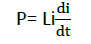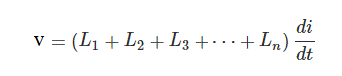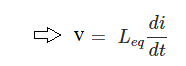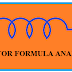## Inductance Formulas and Equations

An inductor is a passive element. The inductor is used in all electrical circuits. We already posted an article about Inductor and Inductance you can read. Today we are going to know all Inductance Formulas and Equations with a detailed explanation. The inductance formula is very very important for us.
So let's start Inductance Formula from basics.

We already know that when the flow of current through a coil is changing with time an EMF is induced in the coil and the induced EMF is proportional to the rate of change of the flowing current with time.

so we can write, Inductance L = V/(di/dt)Now,Here L= Inductance

This is the basic inductance formula. Remember that this formula represents Faraday's law of electromagnetic induction. So when we have the voltage and know the changes of current per time duration we are able to find out the Inductance.

Another formula of inductance is:

L = (μ₀ * N² * A) / l

It is the main core formula to find out the inductance of any coil.

where:

L is the inductance in Henries (H),
μ₀ is the permeability of free space (4π × 10^-7 H/m),
N is the number of turns in the coil,
A is the cross-sectional area of the coil in square meters (m²), and
l is the length of the coil in meters (m).

This formula is derived from the concept of self-inductance, which describes the ability of a coil to generate an electromotive force (EMF) itself due to changes in the magnetic field resulting from current flowing through it. The inductance depends on the geometry of the coil, the number of turns, and the material properties.

The equation of current in the Inductor is,In this formula:

i represents the current in the inductor as a function of time (t).
V is the voltage across the inductor.
L is the inductance of the inductor.

Here, the integral of V with respect to time (∫V dt) represents the area under the voltage-time curve, which gives you the total change in the flux through the inductor. Dividing this by the inductance (L) yields the current in the inductor. Also, note that this formula assumes that the initial current at t=0 is zero. If there is an initial current present, it needs to be taken into account when determining the current using this formula.

The formula of power in Inductor is,

P = Li(di/dt)You can see this equation shows that the power in an inductor depends on the inductance (L) and the rate of change of current (di/dt). So the power in an inductor is often associated with the energy stored in its magnetic field. The power can be positive or negative, depending on whether energy is being stored in the inductor (positive power) or released from the inductor (negative power) at any given time.

The energy-storing formula of Inductor E =E = (1/2)LI^2

In this formula:

E represents the energy stored in the inductor,
L is the inductance of the inductor,
i is the current flowing through the inductor.

The formula shows that the energy stored in an inductor is directly proportional to the square of the current and the inductance.

The Formula of Self-inductance,Here, L= self-inductance
N= No. of turns
l (small L)= length of the wire
A= area of each turnSo we can write,Self Inductance L = (μ₀ * μᵣ * N² * A) / l

This formula shows that the self-inductance of an inductor is directly proportional to the square of the number of turns (N²) and the cross-sectional area (A), while inversely proportional to the length of the wire (l).

### Inductance Formula of Series Inductor circuit:

If a circuit consists 'n' no. of inductors in series then the equivalent inductance of the circuit will be,We know that the voltage drop across in one inductor is,So according to Kirchhoff's Voltage Law, the total voltage drop across the circuit will be,Now we can write,### Inductance Formula of Parallel Inductor circuit:

If 'n' no of inductors are connected in parallel the equivalent inductance will be,As it is a parallel the voltage across each inductor will be the same, but current will be different.
The current in each inductor is,
L1=i1, L2=i2, L3=i3, Ln=in

According to Kirchhoff's Current Law,

The total current               i=i1+i2+i3+.........+in

Now,These are the Inductance Formulas.

All Inductance Formulas and Equations ExplainedReviewed by Author on December 21, 2018 Rating: 5